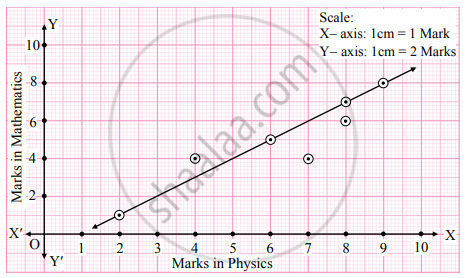# For the following data of marks of 7 students in physics (x) and Mathematics (y), draw scatter diagram and state the type of correlation. x 8 6 2 4 7 8 9 y 6 5 1 4 4 7 8 - Mathematics and Statistics

Diagram

For the following data of marks of 7 students in physics (x) and Mathematics (y), draw scatter diagram and state the type of correlation.

 x 8 6 2 4 7 8 9 y 6 5 1 4 4 7 8

#### Solution

We take marks in Physics on X-axis and marks in Mathematics on Y-axis and plot the points as below.We get a band of points rising from left to right. This indicates the positive correlation between marks in Physics and marks in Mathematics.

Concept: Statistics (Entrance Exam) - Scatter Diagram
Is there an error in this question or solution?